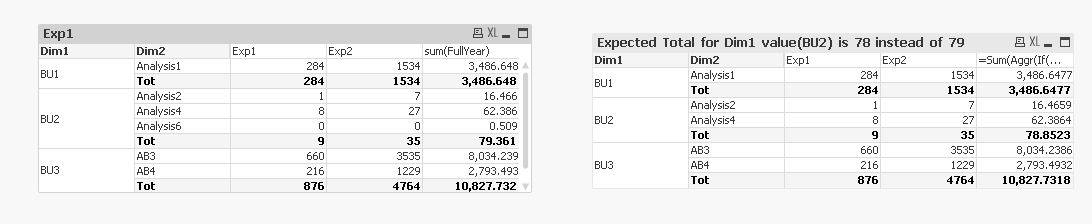# QlikView App Development

Discussion Board for collaboration related to QlikView App Development.

Highlighted
Contributor II

## Pivot Table expression row count

Hello Experts,

I have some problem in partial count of an expression.Chart1 is the default chart of a pivot table. In the third expression the logic is if the 2nd exp value <>0 then only those values should appear. That's fine. But when i apply partial sum that also applies to the missing value. Instead of 78 i am getting the same 79.

QVW attached. !

Tags (3)
1 Solution

Accepted SolutionsMVP

## Re: Pivot Table expression row count

Try this

=Sum(Aggr(If(Sum(Exp2)<>0,

Sum(FullYear)), Dim1, Dim2))3 RepliesMVP

## Re: Pivot Table expression row count

Try this

=Sum(Aggr(If(Sum(Exp2)<>0,

Sum(FullYear)), Dim1, Dim2))Contributor II

## Re: Pivot Table expression row count

Thank You . It worked

Contributor II

## Re: Pivot Table expression row count

I have tried the same. but i missed to write sum here (...Sum(Exp2).

Thanks:)